Web www.handwritingforkids.com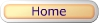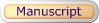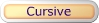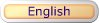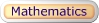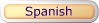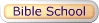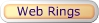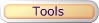### Online Math 3 - Subtraction Math Drill/Test Maker 2 Digits/1 Digit (Vertical)

This online/interactive generator will provides plenty of math drill and practice in subtraction, to boost test scores, improves accuracy and speed to prepare the child/children to meet the Grade 1, Grade 2, and Grade 3 curriculum standards.

This online/interactive math subtraction will create 2 digits by 1 digit (2x1) equations with an options of "No Borrowing", "Borrowing", or "Mixed" (both borrowing and no borrowing) math equations, and timed test. Also, it will automatically graded/scored your results in a printable worksheet. Note: This program will randomly select minuend numbers between 10-99, and subtrahend numbers between 0-9.

Type of online math problems:

• Subtraction - No Borrowing
• Subtraction - Borrowing
• Subtraction - Mixed - Borrowing & No Borrowing
 46 - 4 42minuendsubtrahenddifference

Follow these steps to make your worksheet.
1. Type/enter your full name.
2. Select the number of math problems per page. (Default: 1 - 20)
3. Select Type of Math Problems. (Default: No Borrowing)
4. Do you want a timed-test? (Default: Yes)
5. Click on submit to make your personalized vertical math subtraction worksheet.
6. Option: Page back and click Submit to create a different set of math problems.

Online Math 3 - Subtraction Math Drill/Test Maker
2 Digits / 1 Digit (vertical)

 Name: (optional)

Enter the Number of Math Problems per page:

Default: 1 - 20

Type of Math Problems:

Default: (No Borrowing)

Yes, Timed-Test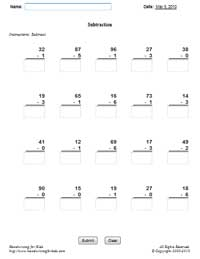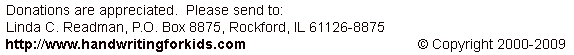Basic Handwriting for Kids Free for Non-Profit Use Ms. Readman, Rockford, IL Revised: